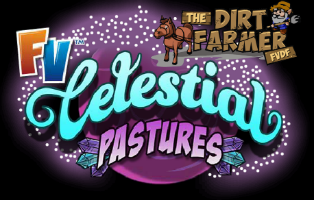# Farmville Celestial Pastures Planet Dirt Farm Crops InfoLike previous farms, Farmville Celestial Pastures comes with  its unique set of new crops to master. Check below for a chart detailing harvest times, coin yields and more. Don’t forget to share this post with your friends if you find it helpful.

Farmville Celestial Pastures Trees

 Crop Cost in Coins Coin Yield XP Gain Time MasteryDazzlers 375 630 1 46 Hours 600+600+600=1800Flava Corn 280 395 1 23 Hours 600+600+600=1800Milky Strawberries 115 350 1 8 Hours 600+600+600=1800Moon Melons 240 350 1 20 Hours 600+600+600=1800Moon Mischief 195 290 1 16 Hours 600+600+600=1800Myne Melon 195 330 1 23 Hours 600+600+600=1800Ooval Tomato 115 175 1 10 Hours 600+600+600=1800Pod Peas 150 225 1 12 Hours 600+600+600=1800Ruzberries 105 160 1 8 Hours 600+600+600=1800Shard Skin Onion 210 305 1 15 Hours and 54 Minutes 600+600+600=1800Solar Flare Bean 225 360 1 23 Hours 600+600+600=1800Space Dust Sugar Cane 265 410 1 23 Hours 600+600+600=1800Star Puff Cotton 215 290 1 12 Hours 1200+2400+3600=7200Star Speckles 385 760 1 69 Hours 600+600+600=1800Stargazer Lilies 200 300 1 15 Hours and 54 Minutes 600+600+600=1800Sun Dipped Pepper 245 350 1 19 Hours and 54 Minutes 600+600+600=1800Sun Fade Barley 165 240 1 14 Hours 600+600+600=1800Sun Fingers 190 285 1 15 Hours and 54 Minutes 600+600+600=1800Supernova Strawberries 110 170 1 9 Hours and 30 Minutes 600+600+600=1800Thuck Wheat 145 220 1 12 Hours 600+600+600=1800Turni Carrot 190 285 1 15 Hours and 54 Minutes 600+600+600= 1800Violet Vein Spinach 175 270 1 14 Hours 600+600+600= 1800White Dwarf Sunflower 285 420 1 23 Hours 600+600+600=1800

Technorati Tags: ,,,,,,,